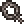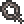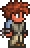••类型 \l!--\n --\g\{\{#vardefine:_typepage\!\{\{#or:\{\{l10n\!item_infobox\!typepage:@@@@\}\}\!\{\{tr\!\{\{ucfirst:@@@@\}\}\!link\ey\}\}\}\}\}\}\l!--\_\n --\g\{\{#vardefine:_typetext\!\{\{#or:\{\{l10n\!item_infobox\!type:@@@@\}\}\!\{\{tr\!\{\{ucfirst:@@@@\}\}\}\}\}\}\}\}\l!--\_\n --\g\{\{nowrap\!class\etag\!\{\{ifexists\!\{\{#var:_typepage\}\}\!\(\(\{\{#var:_typepage\}\}\!\{\{#var:_typetext\}\}\)\)\!\{\{#var:_typetext\}\}\}\}\}\}\l!--\n --\g\l!--\n --\g\{\{#vardefine:_typepage\!\{\{#or:\{\{l10n\!item_infobox\!typepage:@@@@\}\}\!\{\{tr\!\{\{ucfirst:@@@@\}\}\!link\ey\}\}\}\}\}\}\l!--\_\n --\g\{\{#vardefine:_typetext\!\{\{#or:\{\{l10n\!item_infobox\!type:@@@@\}\}\!\{\{tr\!\{\{ucfirst:@@@@\}\}\}\}\}\}\}\}\l!--\_\n --\g\{\{nowrap\!class\etag\!\{\{ifexists\!\{\{#var:_typepage\}\}\!\(\(\{\{#var:_typepage\}\}\!\{\{#var:_typetext\}\}\)\)\!\{\{#var:_typetext\}\}\}\}\}\}\l!--\n --\g 120 需要 1 份掉落自经典专家大师

(武装)冰冻僵尸
(武装)
12%

(大)雨衣僵尸
(大)
12%

(小)雨衣僵尸
(小)
12%

total: 1 row(s)

## 小贴士

• 由于僵尸在血月期间大量生成，脚镣在此事件中相对较容易获得。
• 由于其重铸花费较低，此物品很适合用于获得较优秀的修饰语，如奥秘、暴力、幸运、和护佑。
• 拥有“护佑”修饰语时，它会额外提供 4 防御（总共 5）， 这几乎与整套铜盔甲相当（计入套装奖励，总共 6 防御）。